# Available Fault Current: What is it? (And How To Calculate It)

Contents

## What is Available Fault Current?

Available fault current (AFC) is defined as the largest amount of current available during a fault. It is the maximum amount of current that can be delivered to the electrical equipment under a fault condition. The available fault current is also known as the available short-circuit current.

The term ‘Available Fault Current’ was introduced in the 2011 NFPA 70: National Electric Code (NEC) in section 110.24 (latest version of the code).

According to this section, it is compulsory to mark the maximum amount of available fault current with a date of fault current calculation that was performed.

The rating marked as available fault current is not an equipment rating. But it is the maximum amount of unwanted current that will flow on equipment in case of fault occurs.

The term short-circuit current rating (SCCR) is different from available fault current. For all equipment or circuits must not have SCCR less than the AFC.

The reason behind marking AFC in equipment is that the electrician can take that rating and use it to select the proper equipment rating to comply with other code sections like NEC 110.9 and 110.10.

## Available Fault Current Formula

According to NEC 110.24, labeling of available fault current is necessary. But before calculating the available fault current of equipment within dwellings, we require the rating of available fault current at secondary terminals on the utility transformer feeding said dwelling.

In most cases, the rating of available fault current is provided by the utility and it is labeled at the secondary terminal of the utility transformer.

According to this rating, the available fault current is calculated for all equipment. The calculation for all equipment is different as it depends on the circuit impedance.

Follow the below steps to calculate the available fault current;

1. Find the system voltage (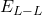)
2. Find the conductor constant (C) from the table
3. Find the length of the service entrance conductor (L)
4. Now, using the above values, calculate the value of the multiplier (M) using the below equations.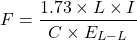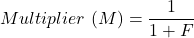1. To find the available fault current at premises, this multiplier (M) is multiplied by the available fault current labeled at the secondary terminal of the utility transformer.

## How to Calculate Available Fault Current

Let’s take an example to understand how to calculate available fault current.

For that we consider a three-phase system having 480V line-line voltage. And the conductor constant C for this system is 13900.

The available fault current at a secondary winding of the utility transformer is 35000A, and the length of the service entrance conductor is 100ft.

EL-L = 480V

C = 13,900

I = 35,000A

L = 100ft

Now, put these values in the above equation.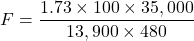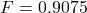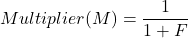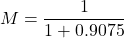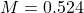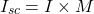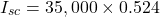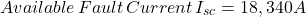## How to Reduce Available Fault Current

In a low-resistance circuit, the current that passes through the circuit increases drastically. And it may damage the circuit. Therefore, we need to use other concepts or equipment to reduce the short circuit current or available fault current.

Mainly there are three methods to reduce available fault current:

1. Increasing cable length
2. Using current limiting reactors
3. Using current limiting devices

### Increase Cable Length

We can reduce the fault current by increasing the length of the cable.

In the above example, we have taken the length of the cable as 100ft. Instead of that, let’s calculate the fault current with a 200ft length cable and other parameters remaining as it is.

By calculating the above steps, you can find the available fault current is 12460A. Hence, it is reduced by increasing the length of the cable.

Observing the relationship between F and L, we can see that they are directly proportional to one another.

### Using Current Limiting Reactor

The reactors can be used at any place in the distribution system to reduce the fault current. However, it will degrade the voltage profile during normal operation. But it is the cheapest method to reduce the fault current.

In most cases, a current limiting reactor is connected in series with the circuit or system.

### Using Current Limiting Devices

This is a non-linear device that is used in two states; conducting (zero resistance) and limiting (high resistance).

When the circuit is operating in normal conditions, this device simply conducts and there is no change in the voltage profile.

But as the current increases, this device increases the resistance in the circuit to limit the magnitude of the fault current.